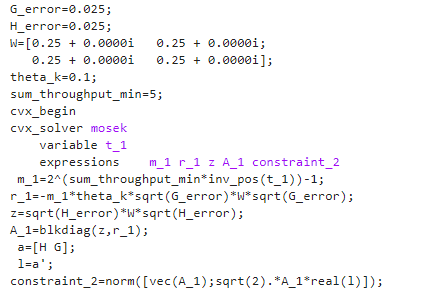# Cannot perform the operation norm( {mixed concave/constant}, 2 )

I am trying to solve minimization problem using CVX. But I got the given error. What could be the reason for this?
Problem：

``````G_error=0.025;
H_error=0.025;
W=[0.25 + 0.0000i   0.25 + 0.0000i;
0.25 + 0.0000i   0.25 + 0.0000i];
theta_k=0.1;
sum_throughput_min=5;
cvx_begin
cvx_solver mosek
variable t_1
expressions    m_1 r_1 z A_1 constraint_2
m_1=2^(sum_throughput_min*inv_pos(t_1))-1;
r_1=-m_1*theta_k*sqrt(G_error)*W*sqrt(G_error);
z=sqrt(H_error)*W*sqrt(H_error);
A_1=blkdiag(z,r_1);
a=[H G];
l=a';
constraint_2=norm([vec(A_1);sqrt(2).*A_1*real(l)]);
``````

Disciplined convex programming error:
Cannot perform the operation norm( {mixed concave/constant}, 2 )

constraint_2=norm([vec(A_1);sqrt(2).A_1real(l)]);

thanks!!`m_1` is convex; Therefore, `r_1` is concave. Therefore `A_1` consists of a mixture of constant (`z`) and concave (1r_11). The DOP rules require the argument of `norm` to be affine, which `A_1` is not.Therefore, CVX does not allow `constraint_2` to be formed.

However, because I don’t know how `constraint_2` is used in your problem, I can’t say for sure that the problem is non-convex. Nevertheless, until you show your problem (which you have not shown it its entirety) is convex, I will assume it is not.

I have no idea what this is, because I don’t know what the optimization (decision) variables are. You say `a` is an optimization variable, but `a` is not in the constraint in the image, unless it is lurking behind the scenes in some relationship you haven’t told us about.

Your first task is to understand what the problem statement s. Copying a problem from a paper or book without understanding what all the symbols, assumptions, and notation are and mean is not a good way to conduct research. Once you understand the problem statement, you then need to prove that the optimization problem is convex. Once you have done that, if you have trouble entering the problem into CVX, you can ask for assistance from forum readers in doing so.

Hi! Mark, I reorganized the problem, I don’t know why r_1 becomes concave so that matlab will report this error, please help me to see this problem.

Disciplined convex programming error:
Cannot perform the operation norm( {mixed concave/constant}, 2 )

constraint_2=norm([vec(A_1);sqrt(2)A_1real(l)]);

`m_1` is not affine. Therefore, `r1` is not affine.Therefore the argument of `norm` is not affine. So you have violated CVX’s rules. You have yet to prove your optimization problem is convex.

In the future, please copy and paste using Preformatted text icon, rather than posting image fo your code.